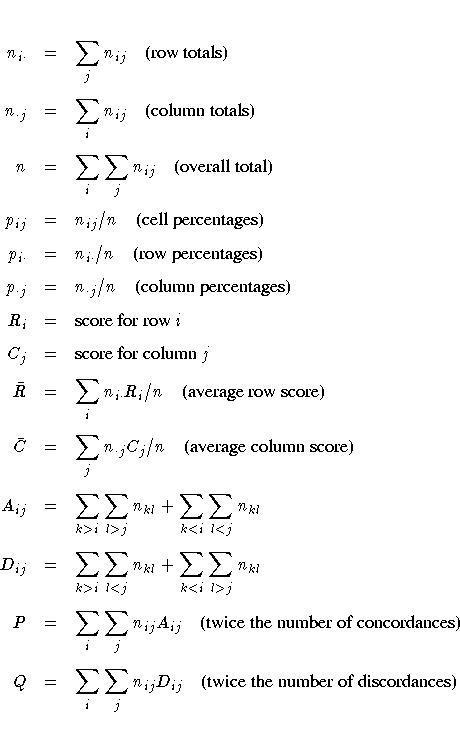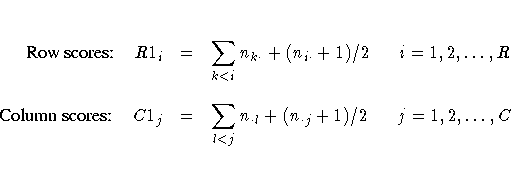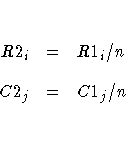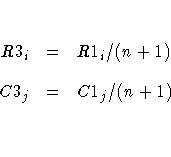The FREQ Procedure

### Definitions and Notation

In this chapter, a two-way table represents the crosstabulation of variables X and Y. Let the rows of the table be labeled by the values Xi, i = 1, 2, ... , R, and the columns by Yj, j = 1, 2, ... , C. Let nij denote the cell frequency in the ith row and the jth column and define the following:#### Scores

PROC FREQ uses scores for the variable values when computing the Mantel-Haenszel chi-square, Pearson correlation, Cochran-Armitage test for trend, weighted kappa coefficient, and Cochran-Mantel-Haenszel statistics. The SCORES= option in the TABLES statement specifies the score type that PROC FREQ uses. The available score types are TABLE, RANK, RIDIT, and MODRIDIT scores. The default score type is TABLE.

For numeric variables, table scores are the values of the row and column levels. If the row or column variables are formatted, then the table score is the internal numeric value corresponding to that level. If two or more numeric values are classified into the same formatted level, then the internal numeric value for that level is the smallest of these values. For character variables, table scores are defined as the row numbers and column numbers (that is, 1 for the first row, 2 for the second row, and so on).

Rank scores, which you can use to obtain nonparametric analyses, are defined byNote that rank scores yield midranks for tied values.

Ridit scores (Bross 1958; Mack and Skillings 1980) also yield nonparametric analyses, but they are standardized by the sample size. Ridit scores are derived from rank scores asModified ridit (MODRIDIT) scores (van Elteren 1960; Lehmann 1975), which also yield nonparametric analyses, represent the expected values of the order statistics for the uniform distribution on (0,1). Modified ridit scores are derived from rank scores as